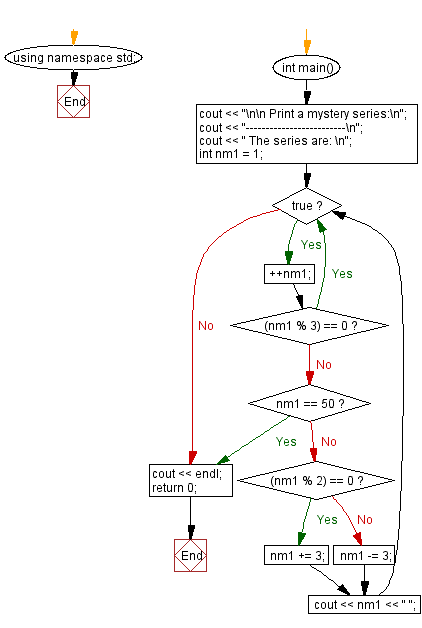﻿ C++ Exercises: Print a mystery series from 1 to 50 - w3resource

# C++ Exercises: Print a mystery series from 1 to 50

## C++ Basic: Exercise-37 with Solution

Write a C++ program to print a mystery series from 1 to 50.

Sample Solution:

C++ Code :

``````#include <iostream>
using namespace std;

int main()
{
cout << "\n\n Print a mystery series:\n";
cout << "-------------------------\n";
cout << " The series are: \n";
int nm1 = 1;
while (true)
{
++nm1;
if ((nm1 % 3) == 0)
continue;
if (nm1 == 50)
break;
if ((nm1 % 2) == 0)
{
nm1 += 3;
}
else
{
nm1 -= 3;
}
cout << nm1 << " ";
}
cout << endl;
return 0;
}
``````

Sample Output:

``` Print a mystery series:
-------------------------
The series are:
5 4 2 7 11 10 8 13 17 16 14 19 23 22 20 25 29 28 26 31 35 34 32 37 41 4
0 38 43 47 46 44 49
```

Flowchart:C++ Code Editor:

What is the difficulty level of this exercise?

﻿

## C++ Programming: Tips of the Day

Why is there no std::stou?

The most pat answer would be that the C library has no corresponding "strtou", and the C++11 string functions are all just thinly veiled wrappers around the C library functions: The std::sto* functions mirror strto*, and the std::to_string functions use sprintf.

Ref: https://bit.ly/3wtz2qA

We are closing our Disqus commenting system for some maintenanace issues. You may write to us at reach[at]yahoo[dot]com or visit us at Facebook